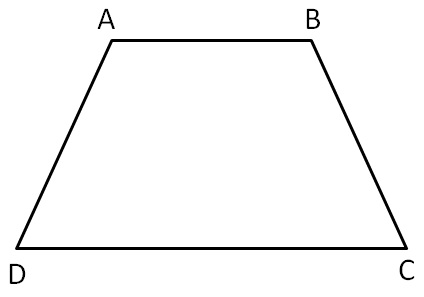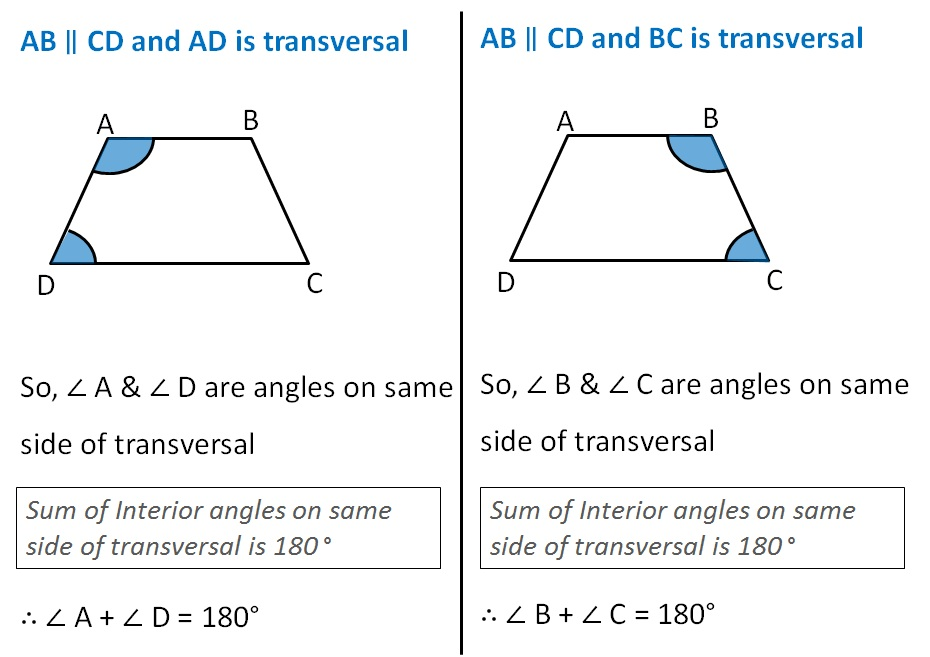Chapter 3 Class 8 Understanding Quadrilaterals
Concept wiseone pair of opposite sides parallel

Here, AB ∥ CD

Thus, sum of adjacent angles in trapezium is 180°

Property:

Adjacent angles of Trapezium are supplementary

i.e. ∠ A + ∠ D = 180°

∠ B + ∠ C = 180°

Let’s look at its proofThus, sum of adjacent angles in trapezium is 180°

Learn in your speed, with individual attention - Teachoo Maths 1-on-1 Class

### Transcript

AB ∥ CD and AD is transversal So, ∠ A & ∠ D are angles on same side of transversal Sum of Interior angles on same side of transversal is 180 ° ∴ ∠ A + ∠ D = 180° AB ∥ CD and BC is transversal AB ∥ CD and BC is transversal So, ∠ B & ∠ C are angles on same side of transversal Sum of Interior angles on same side of transversal is 180 ° ∴ ∠ B + ∠ C = 180° Thus, sum of adjacent angles in trapezium is 180°